Journal DetailsFormat
Journal
eISSN
1891-5469
First Published
01 Jan 2010
Publication timeframe
1 time per year
Languages
EnglishOpen Access

# Frequency dependent rectifier memristor bridge used as a programmable synaptic membrane voltage generator

###### Received: 29 Dec 2012
Journal DetailsFormat
Journal
eISSN
1891-5469
First Published
01 Jan 2010
Publication timeframe
1 time per year
Languages
English

Reasoned by its dynamical behavior, the memristor enables a lot of new applications in analog circuit design. Since some realizations have been shown (e.g. 2007, Hewlett Packard), the development of applications with memristors becomes more and more interesting. Besides applications in neural networks and storage devices, analog memristive circuits also promise further applications. Therefore, this article proposes a frequency dependent rectifier memristor bridge for different purposes, for example, using as a programmable synaptic membrane voltage generator for Spike-Time-Dependent-Plasticity and describes the circuit theory. In this context it is shown that the Picard Iteration is one possibility to analytically solve the system of nonlinear state equations of memristor circuits. An intuitive picture of how a memristor works in a network in general is given as well and in this context some research on the dynamical behavior of a HP memristor should be done. After all it is suggested to use the memristor bridge as a neuron.

#### Keywords

Introduction

The usage of memristors in analog circuit design enablesnew applications. In , an ADC consisting of memristors has been proposed. Other applications are an automatic gain control circuit , programmable analog circuits , an electrical potentiometer  or oscillators [4, 5]. In  memristors are used for basic arithmetic operations. In this paper a frequency-dependent rectifier memristor bridge is presented and therefore, a broad description of memristive systems will be given first.

In general, a memristive system is described by the two equations 

$V(t)=M(x_,I,t)⋅I(t)$$$V\left( t \right)=M\left( \underset{\scriptscriptstyle-}{x},I,t \right)\cdot I\left( t \right)$$$dx_dt=f_(x_,I,t)$$$\frac{\text{d}\underset{\scriptscriptstyle-}{x}}{\text{d}t}=\underset{\scriptscriptstyle-}{f}\left( \underset{\scriptscriptstyle-}{x},I,t \right)$$

where V(t) is the applied voltage and I (t) is the current through the device. $M(x_,I,t)$$M\left( \underset{\scriptscriptstyle-}{x},I,t \right)$is called memristance and correlates voltage and current. Thus, similar to a linear resistor its dimension is Ohm. $x_$$\underset{\scriptscriptstyle-}{x}$is a vector of the dimension n which is given by the number of internal state variables. The dynamics of this vector are specified by the equations (1) and (2).

HP memristor

The HP memristor was realized in 2007 by a team of Hewlett Packard researchers led by Stanley Williams [8, 9]. While this memristor is passive, the latest realization of a memristor is that of an active one on the base of niobium oxide . The HP memristor is made of a titanium dioxide layer which is located between two platinum electrodes. This layer is of the dimension of several nanometers and if an oxygen dis-bonding occurs, its conductance will rise instantaneously. However, without doping, the layer behaves as an isolator. The area of oxygen dis-bonding is referred to as space-charge region and changes its dimension if an electrical field is applied. This is done by a drift of the charge carriers. The smaller the insulating layer, the higher the conductance of the memristor. Also, the tunnel effect plays a crucial role. Without an external influence the extension of the space-charge region do not change. The internal state x is the normed extent of the space-charge region and can be described by the equation

$x:=wD,0≤x≤1,x∈ℝ$$$x:=\frac{w}{D},0\le x\le 1,x\in \mathbb{R}$$

where w is the absolute extent of the space-charge region and D is the absolute extent of the titanium dioxide layer. The memristance can be described by the following equation :

$M(x)=Ron⋅x+Roff⋅(1−x).$$$M\left( x \right)={{\text{R}}_{\text{on}}}\cdot x+{{\text{R}}_{\text{off}}}\cdot \left( 1-x \right).$$

Ron is the resistance of the maximum conducting state and Roff represents the opposite case. The vector containing the internal states of the HP-memristor is one dimensional. For this reason scalar notation is used. The state equation is

$dxdt=f(x,I,t)=μv⋅RonD2⋅IM(t)$$$\frac{\text{d}x}{\text{d}t}=f\left( x,I,t \right)=\frac{{{\mu }_{v}}\cdot {{\text{R}}_{\text{on}}}}{{{D}^{2}}}\cdot {{\text{I}}_{\text{M}}}\left( t \right)$$

where μv is the oxygen vacancy mobility and IM(t) is the current through the device. The following quantizations are used for memristors in this paper .

$Ron=100Ω,μv=10−14m2s⋅VRoff=16kΩ,D=10−8m .$$$\begin{array}{*{35}{l}}{{\text{R}}_{\text{on}}}=100\Omega , & {{\mu }_{v}}={{10}^{-14}}\frac{{{m}^{2}}}{s\cdot V} \\{{\text{R}}_{\text{off}}}=16k\Omega , & D={{10}^{-8}}m\,. \\\end{array}$$

These values are given as an example by the Hewlett Packard Team. The ratio of maximum and minimum value of the memristance can be as large as e.g. 160 .

Window function

The extension of space-charge region is physically limited. Therefore, a window function was established in order to realize a more realistic model which includes saturation. Several shapes of window functions are possible e.g. . In the following, the window function

$fW(x)={(4⋅x⋅(1−x))14,if 0$${{\text{f}}_{\text{W}}}\left( x \right)=\left\{ \begin{array}{*{35}{l}}{{\left( 4\cdot x\cdot \left( 1-x \right) \right)}^{\frac{1}{4}}}, & \text{if}\,0<x<1 \\\,\,\,\,\,\,\,\,\,\,\,\,\,\,\,0, & \text{else} \\\end{array} \right.$$

is used . The current mathematical description is not optimal for numerical calculations. Excluding external influences, in the case of {x ≤ 0 or x ≥ 1} the window function will be 0. Therefore, a state change of the system will not be possible. However, considering VM, the voltage over the memristor, for the two cases x ≤ 0 and VM > 0 or x ≥ 1 and VM < 0, a state change should be possible. The window function results in:

$fW(x,VM)={(4⋅x⋅(1−x))14,if ε0or x≥1−ε and VM<0 0,else${{\text{f}}_{\text{W}}}\left( x,{{\text{V}}_{\text{M}}} \right)=\left\{ \begin{array}{*{35}{l}}{{\left( 4\cdot x\cdot \left( 1-x \right) \right)}^{\frac{1}{4}}}, & \begin{align}& \\ & \text{if}\,\varepsilon <x<1-\varepsilon \\ & \text{or}\,x\le \varepsilon \,and\,{{\text{V}}_{\text{M}}}>0 \\ & \text{or}\,\,x\ge 1-\varepsilon \,and\,{{\text{V}}_{\text{M}}}<0 \\ \end{align} \\\,\,\,\,\,\,\,\,\,\,\,\,\,\,\,0, & \text{else} \\\end{array} \right.

A better stability for numerical simulations is achieved by introducing ε and therefore an extended restriction of definition area. For all simulations in this paper, ε equals 0.02.

Time response of a HP memristor
Change of the internal state

The calculations of this part are done by neglecting the window function. The mathematical description using the window function is exceedingly complicated. The aim is to investigate the time behavior of a HP memristor when connected in series with an AC sine current source IS. Using the state equation (5)

$dxdt=μv⋅RonD2︸=const⋅I0⋅sin(ω⋅t)︸IS=IM,$$$\frac{\text{d}x}{\text{d}t}=\frac{{{\mu }_{v}}\cdot {{\text{R}}_{\text{on}}}}{\underbrace{{{D}^{2}}}_{=const}}\cdot \underbrace{{{I}_{0}}\cdot \sin \left( \omega \cdot t \right)}_{{{\text{I}}_{\text{S}}}={{\text{I}}_{\text{M}}}},$$

dividing of the variables on both sides

$∫x0x0+Δxdx′=const⋅I0⋅∫t0t0+Δtsin(ω⋅t′)dt′,Δt≥0$$$\int_{{{\text{x}}_{0}}}^{{{\text{x}}_{\text{0}}}+\Delta x}{d{x}'=const\cdot {{I}_{0}}\cdot }\int_{{{\text{t}}_{0}}}^{{{\text{t}}_{\text{0}}}+\Delta t}{\sin \left( \omega \cdot {t}' \right)d{t}',\Delta t\ge 0}$$

and performing integration leads to

$Δx=−I0⋅constω⋅(cos(ω⋅(t0+Δt))−cos(ω⋅t0))$$$\Delta x=-\frac{{{I}_{0}}\cdot const}{\omega }\cdot \left( \cos \left( \omega \cdot \left( {{t}_{0}}+\Delta t \right) \right)-cos\left( \omega \cdot {{t}_{0}} \right) \right)$$

whereas x0 := x(t0). At (t0+ Δt) the extension of the space-charge region is (x0+ Δx). In the case of a memristor which is connected in series with a sine current source, the change of the space charge region Δx does not depend on the initial state x0 (see equation (10)). When using a sine voltage source different results are acquired. In this case the state equation is

$dxdt=μv⋅RonD2︸=const⋅V0⋅sin(ω⋅t)M(x)︸IM.$$$\frac{\text{d}x}{\text{d}t}=\frac{{{\mu }_{v}}\cdot {{\text{R}}_{\text{on}}}}{\underbrace{{{D}^{2}}}_{=const}}\cdot \frac{{{V}_{0}}\cdot \sin \left( \omega \cdot t \right)}{\underbrace{M\left( x \right)}_{{{\text{I}}_{\text{M}}}}}.$$

and due to M (x) (linear function), integration of the state equation leads to a quadratic expression. The relevant solution of this quadratic equation for x with t0 = 0 is

$Δx=(r−x0)−(r−x0)2+b⋅(cos(ω⋅Δt)−1)$$$\Delta x=\left( r-{{\text{x}}_{0}} \right)-\sqrt{{{\left( r-{{\text{x}}_{0}} \right)}^{2}}+b\cdot \left( \cos \left( \omega \cdot \Delta t \right)-1 \right)}$$

whereas $r:=RoffRoff−Ron and b:=2⋅V0⋅constω⋅(Roff−Ron).$$r:=\frac{{{\text{R}}_{\text{off}}}}{{{\text{R}}_{\text{off}}}-{{\text{R}}_{\text{on}}}}\,\text{and}\,b:=\frac{2\cdot {{V}_{0}}\cdot const}{\omega \cdot \left( {{\text{R}}_{\text{off}}}-{{\text{R}}_{\text{on}}} \right)}.$Since the change of the space charge region Δx at Δt = 0 has to be zero, the second solution of (12) is irrelevant. Resulting from the disregard of the window function, the usage of equation (12) is limited, since the solution has to be within the physical limits. For one initial state, the reader can proof the results of equation (12) for two voltages with the amplitudes +V0 and −V0. In the case of +V0 the magnitude of Δx after half a period is larger compared to the other case. This means, in the area of lower memristance, the state change performs faster.

In conclusion, Δx depends on the initial state x0, if there is a voltage source in series with the memristor. As shown previously, for a supplied current source it does not. Fig. 1(a) illustrates this insight. Since the memristance is determined by the internal state, the change of the memristance behaves similarly. As seen in Fig. 1(b), for a sine voltage source the change of the memristance depends strongly on the internal memristance, however for a sine current source it does not. Note in the case of a sinusoidal signal, the maximum change of the space charge region Δxmax will be reached at the end of one half period. This is because the direction of the change of the space charge region depends on the algebraic sign of the external signal. Note for calculations and simulations at this chapter, ideal sources were used. When using real sources, the influence of the internal resistances (e.g. Rvoltagesource = 10Ω and Rcurrentsource = 1MΩ) is marginal.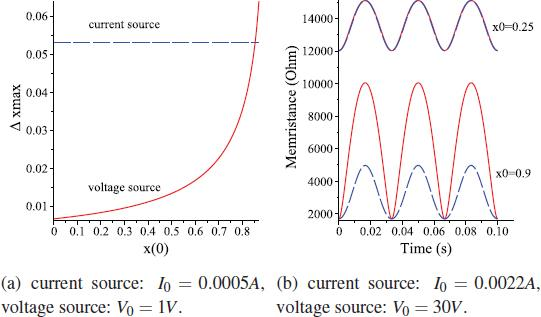Dashed line: supplied sine current source, solid line: supplied sine voltage source, f = 30Hz, (a) Maximal state change xmax dependent on x0, Δt=T2,$\Delta t=\frac{T}{2},$(b) M(x) as a function of t for two different initial states and two source types.
Highest frequency for fully state change

To estimate the dynamical behavior of the HP-memristor in circuits, the frequency fcut for sinusoidal signals is introduced. This is the highest frequency for which a memristor will be able to change from lowest to highest memristance or vice versa. This state change completes exactly at the end of one half period (Δtcut = 0.5 · Tcut ). Note for the used memristor model and same conditions, the required time for changing from a state A to a state B is the same as for the reverse process. fcut depends on the amplitude of the supplied source. Neglecting the window function, the calculations is performed for a single memristor in series with a supplied sine voltage source. This example allows rough estimates for circuits which are more complex. Taking into account that ω equals 2 · π · f and T equals $1f,$$\frac{1}{f},$integration of equation (12) and solving for f with t = 0 leads to

$fcut=1π⋅V0⋅const(Δx22+x0⋅Δx)⋅(Ron−Roff)+Δx⋅Roff$$${{\text{f}}_{\text{cut}}}=\frac{1}{\pi }\cdot \frac{{{V}_{0}}\cdot const}{\left( \frac{\Delta {{x}^{2}}}{2}+{{\text{x}}_{0}}\cdot \Delta x \right)\cdot \left( {{\text{R}}_{\text{on}}}-{{\text{R}}_{\text{off}}} \right)+\Delta x\cdot {{\text{R}}_{\text{off}}}}$$

whereas fcut is direct proportional to the amplitude V0.

Example: Considering the restricted domain of x, the maximal frequency for switching from highest memristance represented by x(t =0)=0.02 to lowest memristance, which implies $x(t=T2)=0.98,$$x\left( t=\frac{T}{2} \right)=0.98,$is calculated. Therefore △x is equal to 0.96. Note that the calculations are made by neglecting the window function. Therefore, for this example the introduced restriction on ε is not really necessary. However, the aim is to have a good comparison to the simulation result including the window function. Calculating by using V0 = 30V leads to fcut ≈ 12.35675Hz. Testing by solving the state equation numerically leads to following results:

$x(t=12⋅f)=0.98 for f=12.35675 Hz$$$x\left( t=\frac{1}{2\cdot f} \right)=0.98\,\text{for}\,f=12.35675\,Hz$$$x(t=12⋅f)=0.9424 for f=12.4 Hz.$$$x\left( t=\frac{1}{2\cdot f} \right)=0.9424\,\text{for}\,f=12.4\,Hz.$$

If the frequency is higher than fcut, saturation would not be reached. Taking the window function into account, the result of solving the state equation by simulation is $x(t=$$x\left( t= \right.$$12⋅f)=0.98$$\left. \frac{1}{2\cdot f} \right)=0.98$for f = 10.75Hz. Therefore the calculations without window function are reasonable for rough estimates. Note for f > fcut, if the frequency is increasing the ratio of maximum and minimum value of the memristance will decrease. Note for a supplied sine current source, the calculation for fcut is also possible (Conversion of Eq. (10)). As an example, for an amplitude $I0=30 VRoff=1.9 mA,$${{I}_{0}}=\frac{30\,V}{{{\text{R}}_{\text{off}}}}=1.9\,mA,$fcut is about 6.2Hz.

Properties

In summary, the value of memristance depends on the previous load . If no current is applied, the internal state will be retained. It follows that a memristor acts like a nonvolatile memory, whereas the range of values is continuous [14, 15]. That is an interesting fact comparable to transistor memory technology. The difference between highest and lowest possible memristance is relatively large . Indeed in the true sense, the memristor is not a switch

$VM1=VS−V10$$${{\text{V}}_{\text{M}}}_{1}={{\text{V}}_{\text{S}}}-{{\text{V}}_{\text{10}}}$$$VM2=−(V10)$$${{\text{V}}_{\text{M2}}}=-\left( {{\text{V}}_{\text{10}}} \right)$$$VM3=−(VS−V20)$$${{\text{V}}_{\text{M3}}}=-\left( {{\text{V}}_{\text{S}}}-{{\text{V}}_{\text{20}}} \right)$$$VM4=V20$$${{\text{V}}_{\text{M4}}}={{\text{V}}_{\text{20}}}$$$VRL=V10−V20$$${{\text{V}}_{\text{RL}}}={{\text{V}}_{\text{10}}}-{{\text{V}}_{\text{20}}}$$

but it could be used for switching operations. Therefore, depending on the direction and the benchmark the memristor passes higher potentials and blocks for lower ones. Subject to the time shift the behavior of the memristor is frequency dependent. For limf→∞ it behaves like a linear resistor  because the change of the internal state is not able to follow the rapid voltage change. For sufficiently small frequencies the nonlinearities are dominating whereas the time shift is directly proportional to the amplitude of the signal.

Memristor bridge circuit

This circuit (Fig. 2) contains four HP memristors, one AC voltage source and one load resistor. By definition, for a positive voltage the memristors M1 and M4 are forward biased, while M2 and M3 are reverse biased. In  and  this circuit has also been presented, but applications differ. While this paper deals with periodic signals and their specifics at different frequencies and initial states, in  pulses are used for synaptic weight programming. In  the focus lies on generation of nth-order harmonics and the effect of frequency doubling by using this circuit.

Mathematical description

At this point the new notation for the memristance

$M(xn):=Mn, n∈ℕ$$$M\left( {{x}_{n}} \right):={{M}_{n}},\,\,n\in \mathbb{N}$$

is established. The voltages

$V10:=V10(x1,x2,x3,x4)=VS⋅Num+M2⋅M3⋅RLDen$$${{V}_{\text{10}}}:={{V}_{10}}\left( {{x}_{1}},{{x}_{2}},{{x}_{3}},{{x}_{4}} \right)={{\text{V}}_{\text{S}}}\cdot \frac{\text{Num}+{{M}_{2}}\cdot {{M}_{3}}\cdot {{\text{R}}_{\text{L}}}}{\text{Den}}$$$V20:=V20(x1,x2,x3,x4)=VS⋅Num+M1⋅M4⋅RLDen$$${{V}_{20}}:={{V}_{20}}\left( {{x}_{1}},{{x}_{2}},{{x}_{3}},{{x}_{4}} \right)={{\text{V}}_{\text{S}}}\cdot \frac{\text{Num}+{{M}_{1}}\cdot {{M}_{4}}\cdot {{\text{R}}_{\text{L}}}}{\text{Den}}$$

are defined from the nodes to ground, whereas the notations

$Num:=(M1+M3+RL)⋅M2⋅M4$$$\text{Num}:=\left( {{M}_{1}}+{{M}_{3}}+{{\text{R}}_{\text{L}}} \right)\cdot {{M}_{2}}\cdot {{M}_{4}}$$$Den:=(M1+M2)⋅(M3⋅M4+M3⋅RL+M4⋅RL) +M1⋅M2⋅(M3+M4).$\begin{align}& \text{Den}:=\left( {{M}_{1}}+{{M}_{2}} \right)\cdot \left( {{M}_{3}}\cdot {{M}_{4}}+{{M}_{3}}\cdot {{\text{R}}_{\text{L}}}+{{M}_{4}}\cdot {{\text{R}}_{\text{L}}} \right) \\ & \,\,\,\,\,\,\,\,\,\,\,\,\,\,\,\,+{{M}_{1}}\cdot {{M}_{2}}\cdot \left( {{M}_{3}}+{{M}_{4}} \right). \\ \end{align}

are used for a better understanding. The structure of the circuit implies a nonlinear system of differential equations of the fourth order. The four state equations are

$dxndt=μv⋅RonD2⋅fW(xn,VMn)⋅VMnM(xn)︸IMn,n≤4.$$$\frac{\text{d}{{x}_{n}}}{\text{d}t}=\frac{{{\mu }_{v}}\cdot {{\text{R}}_{\text{on}}}}{{{D}^{2}}}\cdot {{\text{f}}_{\text{W}}}\left( {{x}_{n}},{{\text{V}}_{\text{M}n}} \right)\cdot \frac{{{\text{V}}_{\text{M}n}}}{\underbrace{M\left( {{x}_{n}} \right)}_{{{\text{I}}_{\text{M}n}}}},n\le 4.$$
Simulation results and functionality

Using these state equations, numerical simulations are possible. For every numerical calculation in this paper the Fehlberg fourth-fifth order Runge-Kutta method is used and RL is 1kΩ.

DC-analysis: Using a DC voltage source, the states change until saturation is reached. The states of M1 and M4 will change in the same direction, which is opposite to M2 and M3. For example, for a positive voltage the memristances M1 and M4 will decrease while M2 and M3 will increase. A DC voltage can be used to set the states of the memristors.

AC-analysis: From this point VS is a sine AC voltage source. Regarding Fig. 3, dependent on the frequency a qualitative difference for the voltage over the load VRL is detectable. For low frequencies (represented by f =0.5Hz in the case of V0 = 30V) the output signal is almost exclusively positive, comparable with a rectifier circuit. Therefore, the frequency of the output signal is twice as high as the one of the input signal. Caused by the time shift of the change of state, which is subject to a HP memristor, there are temporary short negative peaks. Using a high excitation frequency (represented by f = 30Hz for V0 = 30V), no rectifier function is detectable.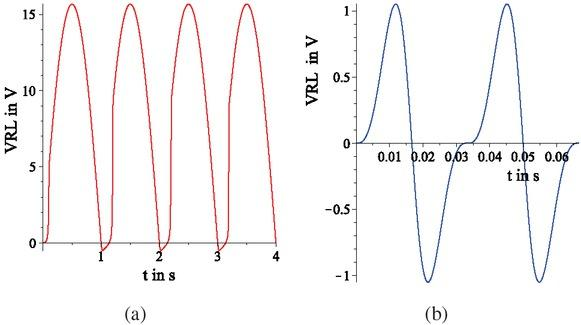Voltage VRL as a function of time t, sine AC voltage source, V0 = 30V, xn(t = 0) = 0.5, (a) f = 0.5Hz, (b) f = 30Hz.

By means of Fig. 4, this frequency selective behavior can be better understood. Note that the asymmetric behavior of the memristances shown in Fig. 4(b) was expected (See equation (12) for a single memristor and its interpretation).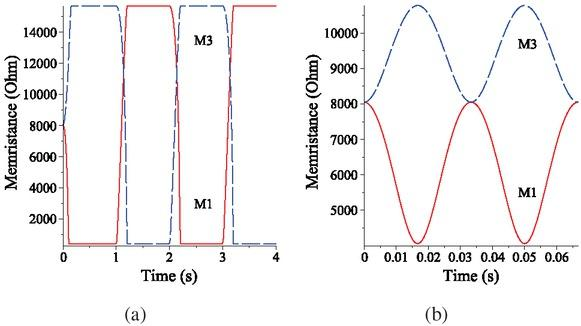Memristances M1 and M3 dependent on time t, sine AC voltage source, V0 = 30V, xn(t = 0) = 0.5, (a) f = 0.5Hz, (b) f = 30Hz.

f ≤fcut: For low frequencies, a complete state change will happen, because the memristors enter saturation. Beginning with an initial value M1 and M4 are decreasing, while M2 and M3 are increasing until saturation is reached. When the sine voltage source reaches its negative half period the process will be reversed until saturation is reached again and so on. During one period there is a change for the relational operator between both memristances. Thus, every half period, the potential in node one is going to be higher than that in node two, which implies that VRL is always going to be positive.

f > fcut: The memristors do not completely switch from lowest to highest memristance. It follows that they do not reach saturation and the memristors will just reach the initial state after one period. Due to this characteristic, the relational operators between M1 and M4 do not change compared to M2 and M3 during one period and the relation of the memristances solely depends on the initial conditions (See Fig. 4(b)). For example, if the initial states are equal in all four memristors, the values of M1 and M4 would be lower than M2 and M3. Thus, VRL would be positive for the first half period, too. At the second half period, the Memristances M2 and M3 keep higher than M4 and M1. Thus, the potential at node “2" is higher than at node “1", which implies that VRL is negative.

There are two reasons why the absolute value for the amplitude of VRL decreases with increasing frequency. On the one hand, for equal initial conditions the maximum potential difference between node “1" and “2" decreases with increasing the frequency. On the other hand, depending on the initial conditions, higher voltage drops across the memristors occur. Note, the maximum amplitude of VRL does not occur at $t=T4.$$t=\frac{T}{4}.$At this time the amplitude of the sine source begins to decrease, but the change of the memristor states is continuing in the same direction, until the sign of VS changes.

The lower the frequencies, the more the circuit behaves like a Graetz circuit, which is shown in Fig. 5(a). Using diodes instead of memristors in the shown configuration, this circuit is used for rectifying. For very high frequencies the circuit behaves like a Wheatstone bridge (see Fig. 5(b)). This can be proven by using the voltage divider. For limRL→∞ it simply is

$VRL=V10−V20=VS⋅M2M1+M2−M4M3+M4,$$${{\text{V}}_{\text{RL}}}={{V}_{10}}-{{V}_{20}}={{\text{V}}_{\text{S}}}\cdot \left( \frac{{{M}_{2}}}{{{M}_{1}}+{{M}_{2}}}-\frac{{{M}_{4}}}{{{M}_{3}}+{{M}_{4}}} \right),$$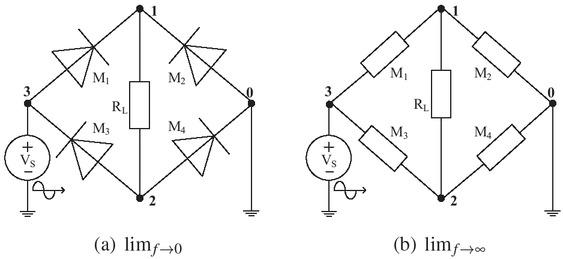Approximate circuit equivalents: (a) Graetz circuit for very low frequencies, (b) Wheatstone circuit for very high frequencies.

and had to be zero for equal resistances, if the conclusion is true. Simulations illustrate that for e.g. f = 50kHz and xn(0) = 0.5 the amplitude of VRL equals 0.5mV, while the ratio between highest and lowest memristance of e.g. M (x1) equals 1.0002.

Using a supply periodic square wave voltage source with

$VS=s gn(sin(ω⋅t)).$$${{\text{V}}_{\text{S}}}=sgn\left( \sin \left( \omega \cdot t \right) \right).$$

is another interesting example. The results for this are shown in Fig. 6. A frequency dependent behavior is also detectable. For high frequencies the voltage over the load resistor is serrated. For low frequencies this voltage is almost constant with negative peaks. The condition that the initial states of all memristors are equal leads to the behavior presented in Fig. 6. But what happens if the circuit is supplied with a periodic square wave voltage and the initial states are not equal? As shown in Fig. 7(a), for a supplied periodic voltage and high frequencies the variation of memristance depends on the initial conditions. Thus, the voltage curve changes from the saw tooth form. As shown in Fig. 7(b), it is possible to create a curve which is similar to synaptic membrane voltages which are used for Spike-Time-Dependent-Plasticity . The amplitude of the voltage varies for different initial conditions, which can be set by a low frequency or DC voltage.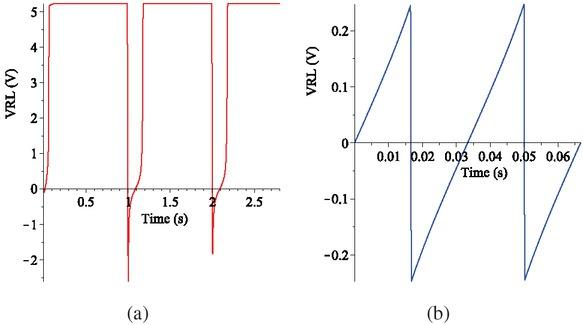VRL dependent on t, supplied periodic square wave voltage source, V0 = 10V, xn(t = 0) = 0.5, (a) f = 0.5Hz, (b) f = 30Hz.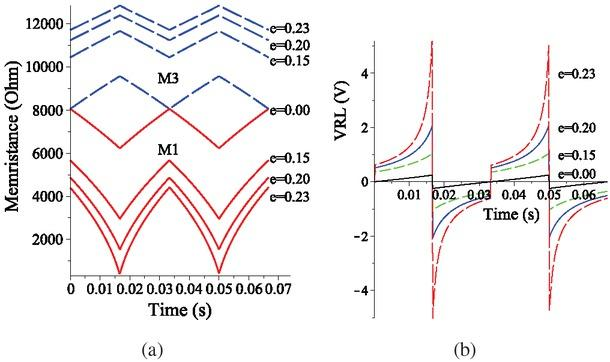System supplied by a periodic square wave voltage source with V0 = 10V, f = 30Hz, x1(0) = x4(0) = 0.5+e, x2(0) = x3(0) = 0.5−e. (a) M1(x) and M3(x) as a function of t for different initial conditions, (b) VRL dependent on t for different initial conditions.
Possible applications

Depending on the frequency, there are two significant types. Fusing a common Graetz circuit and a Wheatstone circuit in one device is one application possibility. Replacing the independent source with a controlled one leads to further application possibilities. The source is controlled by the load voltage and its frequency is variable, keeping in mind, the amplitude of the output signal decreases by increasing the frequency. Because of that, a frequency controlled regulator is conceivable. If the amplitude exceeds a predefined value, the frequency has to increase to prevent a further amplification. Using the circuit as a saw tooth generator is also possible. As mentioned before, the presented circuit could also be used as a programmable synaptic membrane voltage generator for Spike-Time-Dependent-Plasticity.

Synaptic circuit

This chapter will show a connection between the memristor bridge circuit and biological science. In  (electro-osmosis in skin) and  (cell membranes and the Hodgkin-Huxley potential), examples of the usage of memristive devices in biological systems are given. The Hodgkin-Huxley potential itself has been proposed in .

The aim of this paper is to propose using the introduced circuit to model a neuron. For reasons illustrated in Fig. 7, it seems natural to use this circuit to generate output signals which remind of neural impulses. Subsequently in the context of the memristor bridge the terms “neuron" and “cell" are used as synonyms for this memristor bridge circuit. It is assumed that the connection of several memristor bridges models a synaptic circuit (see Fig. 13). In the following, hypotheses on how some biological processes could be applied on the synaptic circuit are given. Periodic square wave signals are not common in the area of synaptic systems, which raises the question: What happens if the signal shown in Fig. 7 is used as input signal instead? Using the voltage waveform shown in Fig. 7 as input voltage of the memristor bridge circuit results in an output waveform as shown in Fig. 8. The observed waveforms are qualitatively similar. Furthermore, it is showed that the amplitude of the output signal is considerably smaller compared to the amplitude of the input signal.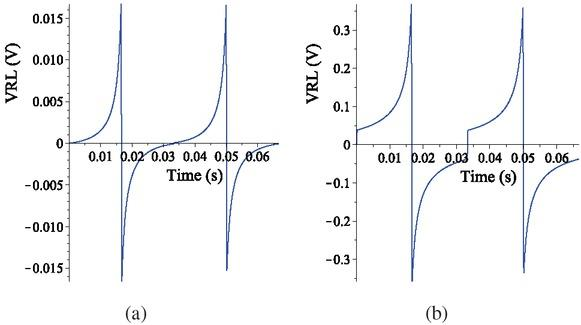Output voltage of the memristor bridge dependent on time. Input voltage is a synaptic impulse as shown in Fig. 7 with e = 0.23. Initial states of this cell: (a) e = 0, (b) e = 0.23.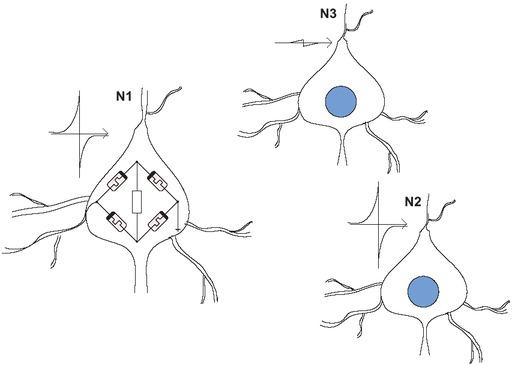Three neurons, N1 and N2 are activated, N3 is not activated (e = 0).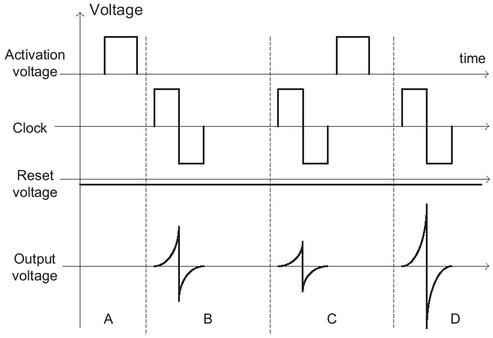Schematic diagram of the voltage curves.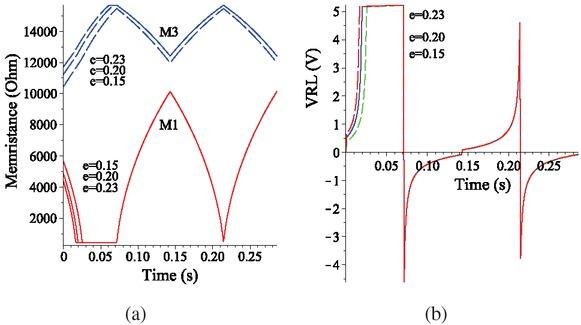System excited by theta waves: periodic square wave voltage source with V0 = 10V, f = 7Hz, x1(0) = x4(0) = 0.5+e, x2(0) = x3(0) = 0.5−e. (a) M1(x) and M3(x) dependent on t for different initial conditions, (b) VRL dependent on t for different initial conditions.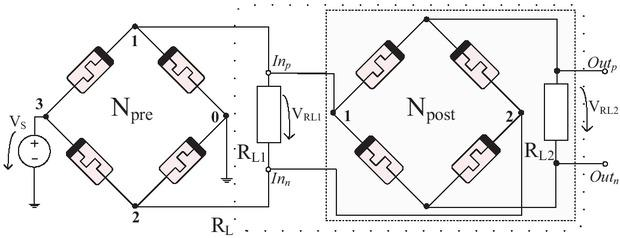Schematic of two combined cells.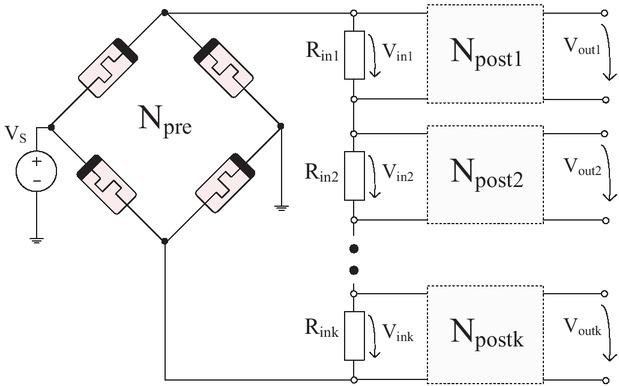Schematic of a presynaptic neuron which is connected to several postsynaptic neurons.

As illustrated in Fig. 7 and 8, it seems, the stronger the initial states of the two memristor pairs differ from each other the larger the amplitude of the output signal becomes. In fact, it strongly depends on whether a pair of memristors (e.g. M1 and M4) reaches a state of low memristance or not (see equations (20) and (21)). For that reason, the output voltage waveform for a set of initial states e.g. x1(0), x4(0) = 0.73 and x2(0), x3(0) = 0.27 compared to an other set x1(0), x4(0) = 0.73 and x2(0), x3(0) = 0.33 is similar. In order to monitor that the initial states differ by the same value from 0.5, the parameter e was introduced. One possibility to set the initial states in the desired way is to apply a DC voltage with a low amplitude over a short time. For example, when applying a voltage of 0.6V and beginning from the initial states xn =0.5, after 0.5s the states x1(0.5s) = x4(0.5s) = 0.73 and x2(0.5s) = x3(0.5s) = 0.33 will be reached (RL = 1KΩ). As expected and discussed before, the state change in direction of lower memristance is faster compared to the direction of higher memristance. However, assuming a symmetric state change and therefore using e for a better description will implicate similar results.

Learning

The state of the whole circuit can be set by applying a DC voltage. If the initial states of all memristors are 0.5, the circuit is not activated. In the other case (e ≠ 0) the circuit is activated. As shown in Fig. 7, the higher e becomes the higher are the peaks generated by the circuit. This is similar to a neuron activated by an external impulse.

Hebbian theory

This theory  suggests that “when an axon of cell A is near enough to excite a cell B and repeatedly or persistently takes part of firing it", the connection between both will improve and signal transmission will become more effective.

Using this theory on the memristor bridge, if cell A and cell B are activated it implies that there is a connection between both. In order to that there is a growth process, the activation has to become larger. A possible circuitry is shown in Fig. 13 and described in the following.

Alternative learning process

If there are two or more neurons activated at the same time, this could be seen as neural learning process. For example, in Fig. 9 neuron N1 is activated by “information 1" and neuron N2 is activated by “information 2". N3 is not included in the learning process. When stimulating all three cells using a clock signal, N1 and N2 are going to fire while the reaction of N3 is marginal. The clock signal can be created by a central pulse generator similar to the thalamus in biological brains . Furthermore, decentralized stimuli generated by neurons connected to neurons of interest can be used. The waveform of the clock signal could be a square wave for technical circuits or, if a stronger reference to biological systems is desired, synaptic impulses.

If two or more neurons are activated and fire at the same phase and frequency, a physical connection could be established. It is conceivable for this connection to increase in strength as the amplitude of the impulses increase. Furthermore, it is essential that several impulses are necessary in order to form a permanent connection. Starting from a non-activated state, by activating a neuron through information e takes for example a value of 0.15. This state decreases over time. To represent this continuous change, a small negative voltage can be applied. Thus, information that should be stored has to be repeated. In Fig. 10 an example of an activation process for a single neuron is given. In phase A the neuron is activated. In B the neuron is stimulated and responses a synaptic impulse. Due to the constant negative voltage, the excitation during phase C leads to a smaller synaptic impulse. Due to the repeated activation at the end of phase C, the response of the circuit is higher in phase D.

Non-REM sleep

The Non-REM sleep is characterized by high amplitudes and low frequencies . It consists of four stages, which this paper will not further describe. The Electroencephalography (EEG) of a human brain shows among other things that there are different rhythms during one day. Beta rhythms have a spectrum from 13Hz up to 30Hz and signalize an active cortex region. In the state of calmness, the EEG shows alpha rhythms (8−12Hz). In stage one of the Non-REM sleep theta waves (4−7Hz) occur, while in stage three delta waves (up to 4Hz) occur. Delta waves characterize the slow-wave sleep phase and have a high amplitude.

In the case of the memristor bridge it is assumed that, for example, the operating mode shown in Fig. 7 is the state of awaking. Relative to this, the frequency of the theta rhythm is lower. The frequency of the delta waves is lower than that of the theta rhythm.

Theta rhythm

As shown in Fig. 11 (a), if the circuit is excited by a lower frequency, which is represented by 7Hz in accordance with theta waves, the memristors are going into saturation during the first half a period. When the sign of the supplied voltage changes, the state changes of all saturated memristors start at the same value. Thus, the waveform of the output voltage after a half a period does not depend on the initial states (see Fig. 11 b).

Interpretation of this results for the Non-REM sleep: One function of the theta rhythm phase could be the alignment of excited neurons. Dependent on the frequency, neurons with a lower state of stimulation or unstimulated neurons do not enter saturation. This could be useful for learning processes.

Delta rhythm

If the frequency is smaller than that of the theta rhythm, the behavior of the circuit differs. In accordance with delta waves, as an example the frequency is set to 2Hz. The memristors are going into saturation. However, if more time is available for the state changes, the memristors will change from state of highest memristance to lowest memristance and vice versa. For that reason, a crossing between the memristances as shown in Fig. 4 is detectable. Assuming this cross point represents the not activated state, it is possible to reset all neurons to this state. For a supplied periodic square wave voltage with V0 = 10V and f = 2Hz, the internal states of the memristors are 0.29 at the cross point. Using these as initial states during a voltage with higher frequencies is supplied (e.g. f = 30Hz, V0 = 10V), the amplitude of the output voltage is 0.08V, which is marginal in relation to the output voltages shown in Fig. 7. Thus, this state of the circuit (xn(t = 0) = 0.29) could be seen as a not activated state.

Interpretation of this results for the Non-REM sleep: One function of the delta rhythm phase can be seen as the resetting of all neurons to the same not activated state. The possibility of changing between activation and non-activation of all neurons together can be seen as working storage. Thus, the delta rhythm phase can be used to refresh this storage.

Neural circuitry

The aim of this section is to suggest, how the physical connection could be realized in circuitry. As shown in Fig. 12, the cell N post is connected in parallel with the resistor RL1. Thus, the cell Npost is excited with the voltage VRL1, which can be calculated by equations (20) and (21). RL is calculated by the resistance of RL1 in parallel with the total memristance of the cell Npost . This fact leads to

$RL(x1,x2,x3,x4)=RL1⋅MN post(x1,x2,x3,x4)RL1+MN post(x1,x2,x3,x4).$$${{R}_{L}}\left( {{x}_{1}},{{x}_{2}},{{x}_{3}},{{x}_{4}} \right)=\frac{{{R}_{L1}}\cdot {{M}_{N\,post}}\left( {{x}_{1}},{{x}_{2}},{{x}_{3}},{{x}_{4}} \right)}{{{R}_{L1}}+{{M}_{N\,post}}\left( {{x}_{1}},{{x}_{2}},{{x}_{3}},{{x}_{4}} \right)}.$$

RL depends on four initial states and is not constant. Due to this the qualitative voltage waveform of VRL1 perhaps changes compared to the in Fig. 7 shown waveforms, for example. Simulations show that with a single memristor used as RL, similar waveforms can be generated. Anyway, in parallel connections the lowest resistance (respectively memristance) is dominating. For example, if the difference between two resistances is given by the factor 10, it is assumed that the summand of lower resistance can be neglected in the denominator of equation (27). Thus, the total resistance equates almost the lower one. For that reason possible values of the total memristance of the memristor bridge circuit are of interest. Using the delta-star transformation and equation (4), the total memristance for one cell can be calculated. The state of non activation of the cell (x1 = x2 = x3 = x4 = 0.5) and the state of saturation (x1 = x4 = 1 and x2 = x3 = 0) are tested. Using RL2 = 1kΩ, the total memristance of the first state is 8.05kΩ and that of the second one is 1.07kΩ. For example, if RL1 equals 100 Ω, the influence of MNpost (x1, x2, x3, x4) is marginal. Note the lower RL, the lower is the amplitude of the output signal, however, the waveform is similar.

Neurons are rather connected with several other neurons than with only one neuron. Regarding Fig. 12 and assuming that RL1 is split into several resistors with lower resistance, connections with more than one cell are possible. This is shown in Fig. 13. The resistors are the connections for further cells and could be compared to axons. For example, if ten resistors with 100Ω are connected in series, the total resistance is 1kΩ. Postsynaptic neurons are simultaneous presynaptic neurons for subsequent cells. Due to this fact this splitting also could be done for RL2, whereas the total resistance is 1kΩ too. For that reason, the values of the given example with RL1 = 100Ω and RL2 = 1kΩ are useful. Note that the voltages over the single resistors (e.g. Rin1 in Fig. 13) are given by the voltage divider. In accordance with the Hebbian theory, if the cell Npre is activated, it means that the connection to all postsynaptic cells is improved. This is a precondition that there are detectable signals at the outputs of the postsynaptic cells. In addition, if a postsynaptic cell is activated too (e.g. Npost1), the amplitude of that output voltage becomes higher compared to the other postsynaptic cells. This implies that the connection between both cells became stronger. The question is, how the activation of the cells can be done automatically by firing of neurons in this circuit itself.

Picard Iteration

At this point, the Picard Iteration is introduced as a possibility to solve common memristive systems analytically. One advantage related to e.g. the Volterra-series expansion is that the Picard Iteration converges more rapidly. This chapter investigates the possibility of applying this iteration to memristive systems by performing on the memristor bridge circuit. First, a general description of the Picard Iteration is given. A nonlinear, dynamical system with

$dxdt=f(t,x(t)), x(t0)=x0$$$\frac{\text{d}x}{\text{d}t}=f\left( t,x\left( t \right) \right),\,\,x\left( {{t}_{0}} \right)={{x}_{0}}$$

is given. The iteration is given by

$x[k+1]:=x0+∫t0tf(t′,x[k](t′))⋅dt′, t∈{t0,t0+ε} .$$${{x}^{\left[ k+1 \right]}}:={{x}_{0}}+\int_{{{t}_{0}}}^{t}{f\left( {t}',{{x}^{\left[ k \right]}}\left( {{t}'} \right) \right)\cdot \text{d}{t}',\,\,t\in \left\{ {{t}_{0}},{{t}_{0}}+\varepsilon \right\}}\,.$$

whereas k denotes the order of the iterative steps.

In the case of the memristor bridge circuit, x(t) is the normalized extent of the space-charge region. The iteration is performed without considering the window function. The window function suppresses changes of the state equation within the marginal area of the memristor. Therefore the window function uses an argument of the same iteration step. This leads to a recursive mathematical expression and shows one weakness of the Picard Iteration. The aim is to perform the iteration only for high frequencies which prevent the memristors from reaching saturation. Thus, the window function can be neglected. It has to be noted that by setting the initial conditions the internal states stay within the domain. Attention has to be paid to the case x > 1 due to the memristance would become negative. Equation (13) is helpful for rough estimates. The frequency has to be greater than fcut.

For memristive systems using the HP memristor model, the Picard Iteration is performed by

$xn[k+1]:=x0+∫t0tμv⋅RonD2⋅VMn [k]M(xn[k])⋅dt′, t∈{t0,t0,+ε}$$$x_{n}^{\left[ k+1 \right]}:={{x}_{0}}+\int_{{{t}_{0}}}^{t}{\frac{{{\mu }_{v}}\cdot {{\text{R}}_{\text{on}}}}{{{D}^{2}}}}\cdot \frac{\text{V}_{\text{M}n}^{\,\,\,\,\left[ k \right]}}{M\left( x_{n}^{\left[ k \right]} \right)}\cdot \text{d}{t}',\,\,t\in \left\{ {{t}_{0}},{{t}_{0}},+\varepsilon \right\}$$

with $VMn[k]=VMn(t,x1[k],x2[k],…,xn[k]).$${{\text{V}}_{\text{M}}}_{n}^{\left[ k \right]}={{\text{V}}_{\text{M}n}}\left( t,x_{1}^{\left[ k \right]},x_{2}^{\left[ k \right]},\ldots ,x_{n}^{\left[ k \right]} \right).$

Similar to chapter “Memristor bridge circuit" the used notation for the memristance $M(xn[k]) is Mn[k]$$M\left( x_{n}^{\left[ k \right]} \right)\,\,\text{is}\,M_{n}^{\left[ k \right]}$with n, k ∈ N. $Num[k]$$\text{Nu}{{\text{m}}^{\left[ k \right]}}$and $Den[k]$$\text{De}{{\text{n}}^{\left[ k \right]}}$is established as well. The only difference to their analogons is the usage of $M(xn[k])$$M\left( x_{n}^{\left[ k \right]} \right)$instead of M (xn).

1st iteration step: Using the initial states $xn=xn(0),dxndt=$$x_{n}^{\left[ 1 \right]}={{x}_{n}}\left( 0 \right),\frac{\text{d}x_{n}^{\left[ 1 \right]}}{\text{d}t}=$0 as arguments for the first iteration step, then the equations for the currents are

$IM1=VS⋅1M1⋅(1−Num+M2⋅M3⋅RLDen)︸MR1$$${{\text{I}}_{\text{M}}}_{1}^{\left[ 1 \right]}={{\text{V}}_{\text{S}}}\cdot \underbrace{\frac{1}{M_{1}^{\left[ 1 \right]}}\cdot \left( 1-\frac{\text{Nu}{{\text{m}}^{\left[ 1 \right]}}+M_{2}^{\left[ 1 \right]}\cdot M_{3}^{\left[ 1 \right]}\cdot {{\text{R}}_{\text{L}}}}{\text{De}{{\text{n}}^{\left[ 1 \right]}}} \right)}_{{{\text{M}}_{\text{R}}}_{1}^{\left[ 1 \right]}}$$$IM2=VS⋅1M2⋅Num+M2⋅M3⋅RLDen︸MR2$$${{\text{I}}_{\text{M}}}_{2}^{\left[ 1 \right]}={{\text{V}}_{\text{S}}}\cdot \underbrace{\frac{1}{M_{2}^{\left[ 1 \right]}}\cdot \frac{\text{Nu}{{\text{m}}^{\left[ 1 \right]}}+M_{2}^{\left[ 1 \right]}\cdot M_{3}^{\left[ 1 \right]}\cdot {{\text{R}}_{\text{L}}}}{\text{De}{{\text{n}}^{\left[ 1 \right]}}}}_{{{\text{M}}_{\text{R}}}_{2}^{\left[ 1 \right]}}$$$IM3=VS⋅1M3⋅(1−Num+M1⋅M4⋅RLDen)︸MR3$$${{\text{I}}_{\text{M}}}_{3}^{\left[ 1 \right]}={{\text{V}}_{\text{S}}}\cdot \underbrace{\frac{1}{M_{3}^{\left[ 1 \right]}}\cdot \left( 1-\frac{\text{Nu}{{\text{m}}^{\left[ 1 \right]}}+M_{1}^{\left[ 1 \right]}\cdot M_{4}^{\left[ 1 \right]}\cdot {{\text{R}}_{\text{L}}}}{\text{De}{{\text{n}}^{\left[ 1 \right]}}} \right)}_{{{\text{M}}_{\text{R}}}_{3}^{\left[ 1 \right]}}$$$IM4=VS⋅1M4⋅Num+M1⋅M4⋅RLDen︸MR4$$${{\text{I}}_{\text{M}}}_{4}^{\left[ 1 \right]}={{\text{V}}_{\text{S}}}\cdot \underbrace{\frac{1}{M_{4}^{\left[ 1 \right]}}\cdot \frac{\text{Nu}{{\text{m}}^{\left[ 1 \right]}}+M_{1}^{\left[ 1 \right]}\cdot M_{4}^{\left[ 1 \right]}\cdot {{\text{R}}_{\text{L}}}}{\text{De}{{\text{n}}^{\left[ 1 \right]}}}}_{{{\text{M}}_{\text{R}}}_{4}^{\left[ 1 \right]}}$$

2nd iteration step:

$dxndt=±const⋅V0⋅sin(ω⋅t)⋅MRn$$$\frac{\text{d}x_{n}^{\left[ 2 \right]}}{\text{d}t}=\pm const\cdot {{V}_{0}}\cdot \sin \left( \omega \cdot t \right)\cdot {{\text{M}}_{\text{R}}}_{n}^{\left[ 1 \right]}$$

Separating the variables in this equation leads to

$∫xnxndx′=±const⋅V0⋅MRn⋅∫0tsin(ω⋅t′)dt′$$$\int_{x_{n}^{\left[ 1 \right]}}^{x_{n}^{\left[ 2 \right]}}{\text{d}{x}'}=\pm const\cdot {{V}_{0}}\cdot {{\text{M}}_{\text{R}}}_{n}^{\left[ 1 \right]}\cdot \int_{0}^{t}{\sin \left( \omega \cdot {t}' \right)\text{d}{t}'}$$

and integration results in

$xn=xn±const⋅V0ω⋅MRn⋅(1−cos(ω⋅t))$$$x_{n}^{\left[ 2 \right]}=x_{n}^{\left[ 1 \right]}\pm \frac{const\cdot {{V}_{0}}}{\omega }\cdot {{\text{M}}_{\text{R}}}_{n}^{\left[ 1 \right]}\cdot \left( 1-\cos \left( \omega \cdot t \right) \right)$$

whereas for $x1, x4$$x_{1}^{\left[ 2 \right]},\,\,x_{4}^{\left[ 2 \right]}$the sign is positive and for $x2, x3$$x_{2}^{\left[ 2 \right]},\,\,x_{3}^{\left[ 2 \right]}$it is negative. For this circuit for the second step the Picard Iteration gives a good quality solution (regarding Fig. 14). Therefore no further iteration steps should be performed. The voltage over the load resistor can be solved with

$VRL=VS⋅RLDen⋅(M2⋅M3−M1⋅M4).$$${{\text{V}}_{\text{RL}}}^{\left[ 2 \right]}={{\text{V}}_{\text{S}}}\cdot \frac{{{\text{R}}_{\text{L}}}}{\text{De}{{\text{n}}^{\left[ 2 \right]}}}\cdot \left( M_{2}^{\left[ 2 \right]}\cdot M_{3}^{\left[ 2 \right]}-M_{1}^{\left[ 2 \right]}\cdot M_{4}^{\left[ 2 \right]} \right).$$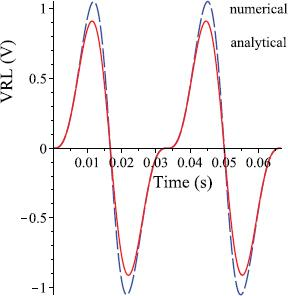VRL dependent on time t, analytical and numerical solution.

Blue dashed line: numerical solution (Fehlberg fourth-fifth order Runge-Kutta method).

Red solid line: analytical solution by Picard Iteration (second iteration step)

Valid for both simulations:$x1=x2=x3=x4=0.5$$x_{1}^{\left[ 1 \right]}=x_{2}^{\left[ 1 \right]}=x_{3}^{\left[ 1 \right]}=x_{4}^{\left[ 1 \right]}=0.5$and V0 = 30V, f = 30Hz

Circuit with similar behavior consisting of two memristors

This circuit (shown in Fig. 15) behaves similar to the memristor bridge, if the applied voltages satisfies three conditions: Amplitude and frequency are the same and the phase is shifted by 180 degrees. The structure of the circuit implies a

nonlinear system of differential equations of the second order whereas the state equations are

$dx1dt=μv⋅RonD2⋅fW(x1,+VM1)⋅(+VS)⋅2⋅RL+M2Den2$$$\frac{\text{d}{{x}_{1}}}{\text{d}t}=\frac{{{\mu }_{v}}\cdot {{\text{R}}_{\text{on}}}}{{{D}^{2}}}\cdot {{\text{f}}_{\text{W}}}\left( {{x}_{1}},+{{\text{V}}_{\text{M1}}} \right)\cdot \left( +{{\text{V}}_{\text{S}}} \right)\cdot \frac{2\cdot {{\text{R}}_{\text{L}}}+{{M}_{2}}}{\text{De}{{\text{n}}_{2}}}$$$dx2dt=μv⋅RonD2⋅fW(x2,+VM2)⋅(+VS)⋅−2⋅RL−M1Den2$$$\frac{\text{d}{{x}_{2}}}{\text{d}t}=\frac{{{\mu }_{v}}\cdot {{\text{R}}_{\text{on}}}}{{{D}^{2}}}\cdot {{\text{f}}_{\text{W}}}\left( {{x}_{2}},+{{\text{V}}_{\text{M2}}} \right)\cdot \left( +{{\text{V}}_{\text{S}}} \right)\cdot \frac{-2\cdot {{\text{R}}_{\text{L}}}-{{M}_{1}}}{\text{De}{{\text{n}}_{2}}}$$

with

$Den2:=M(x2)⋅RL+M(x1)⋅RL+M(x1)⋅M(x2) .$$$\text{De}{{\text{n}}_{2}}:=M\left( {{x}_{2}} \right)\cdot {{\text{R}}_{\text{L}}}+M\left( {{x}_{1}} \right)\cdot {{\text{R}}_{\text{L}}}+M\left( {{x}_{1}} \right)\cdot M\left( {{x}_{2}} \right)\,.$$

VRL is given by the equation

$VRL=RL⋅VS1⋅M(x2)+VS2⋅M(x1)Den2.$$${{\text{V}}_{\text{RL}}}={{\text{R}}_{\text{L}}}\cdot \frac{{{\text{V}}_{\text{S1}}}\cdot M\left( {{x}_{2}} \right)+{{\text{V}}_{\text{S2}}}\cdot M\left( {{x}_{1}} \right)}{\text{De}{{\text{n}}_{2}}}.$$

For this circuit, the frequency selective behavior is detectable. This circuit can also be used as a programmable generator for synaptic impulses. In general, under the same conditions, the amplitude of the output signal is higher compared to the output signal of the other circuit. If it is possible to grip an input signal and its inversion, the usage of this circuit would reduce the complexity by two memristors in comparison to the preceding circuit. Perhaps this circuit could be used to synchronize two synaptic impulses which are phase shifted by 180 degrees.

Conclusions

In this paper two circuits consisting of HP memristors were presented, for those a frequency selective behavior was detectable. In contrast to high frequencies, operation at low frequencies results in a behavior similar to that of a rectifier. This functional change with frequency is caused by the delay when changing the internal states through an external source. To estimate the dynamical behavior of circuits, the time behavior of a single memristor in series with a periodic source was investigated. The configuration of the circuits leads to a nonlinear system of differential equations which describes the internal states. Using the Picard Iteration is one possibility to solve this system analytically. The frequency selective behavior can be used to realize two modes in one circuit (Graetz and Wheatstone circuit). Other applications are a frequency controlled regulator or a programmable synaptic membrane voltage generator for Spike-Time-Dependent-Plasticity. The behavior of the circuit suggests that the circuit could be used as a neuron. For that reason a synaptic circuit is given as well. In this context it is suggested how a learning process could be applied on this synaptic circuit. Additional, assumptions on the functionality of the Non-REM sleep were made.Dashed line: supplied sine current source, solid line: supplied sine voltage source, f = 30Hz, (a) Maximal state change xmax dependent on x0, Δt=T2,$\Delta t=\frac{T}{2},$(b) M(x) as a function of t for two different initial states and two source types.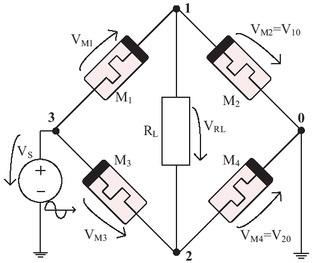Schematic of the memristor bridge circuit.Voltage VRL as a function of time t, sine AC voltage source, V0 = 30V, xn(t = 0) = 0.5, (a) f = 0.5Hz, (b) f = 30Hz.Memristances M1 and M3 dependent on time t, sine AC voltage source, V0 = 30V, xn(t = 0) = 0.5, (a) f = 0.5Hz, (b) f = 30Hz.Approximate circuit equivalents: (a) Graetz circuit for very low frequencies, (b) Wheatstone circuit for very high frequencies.VRL dependent on t, supplied periodic square wave voltage source, V0 = 10V, xn(t = 0) = 0.5, (a) f = 0.5Hz, (b) f = 30Hz.System supplied by a periodic square wave voltage source with V0 = 10V, f = 30Hz, x1(0) = x4(0) = 0.5+e, x2(0) = x3(0) = 0.5−e. (a) M1(x) and M3(x) as a function of t for different initial conditions, (b) VRL dependent on t for different initial conditions.Output voltage of the memristor bridge dependent on time. Input voltage is a synaptic impulse as shown in Fig. 7 with e = 0.23. Initial states of this cell: (a) e = 0, (b) e = 0.23.Three neurons, N1 and N2 are activated, N3 is not activated (e = 0).Schematic diagram of the voltage curves.System excited by theta waves: periodic square wave voltage source with V0 = 10V, f = 7Hz, x1(0) = x4(0) = 0.5+e, x2(0) = x3(0) = 0.5−e. (a) M1(x) and M3(x) dependent on t for different initial conditions, (b) VRL dependent on t for different initial conditions.Schematic of two combined cells.Schematic of a presynaptic neuron which is connected to several postsynaptic neurons.VRL dependent on time t, analytical and numerical solution.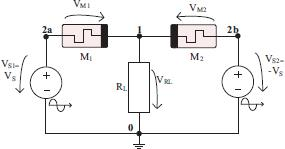Schematic of the circuit with two memristors.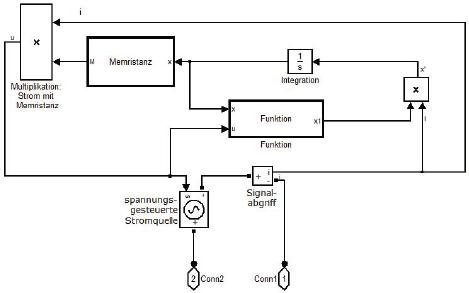Implementation of the HP memristor in Matlab Simulink

Pershin YV, Sazonov E, Di Ventra M. Analog-to-Digital and Digital-to-Analog Conversion with Memristive Devices. Arxiv preprint. 2011;ArXiv: 1111.2903.PershinYVSazonovEDiVentra MAnalog-to-Digital and Digital-to-Analog Conversion with Memristive DevicesArxiv preprint2011ArXiv: 1111.2903Search in Google Scholar

Wey TA, Jemison WD. An automatic gain control circuit with TiO2 memristor variable gain amplifier. In: NEWCAS Conference (NEWCAS), 2010 8th IEEE International. IEEE; 2010. p. 49–52.WeyTAJemisonWDAn automatic gain control circuit with TiO2 memristor variable gain amplifierIn: NEWCAS Conference (NEWCAS)20108th IEEE International. IEEE2010. p495210.1007/s10470-012-9860-5Search in Google Scholar

Pershin YV, Di Ventra M. Practical approach to programmable analog circuits with memristors. Arxiv preprint. 2009;ArXiv: 0908.3162.PershinYVDiVentra MPractical approach to programmable analog circuits with memristorsArxiv preprint2009ArXiv: 0908.316210.1109/TCSI.2009.2038539Search in Google Scholar

Bahgat A, Salama K. Memristor-based mono-stable oscillator. Arxiv preprint. 2012;ArXiv: 1207.0847.BahgatASalamaKMemristor-based mono-stable oscillatorArxiv preprint2012ArXiv: 1207.0847Search in Google Scholar

Talukdar A, Radwan A, Salama K. A memristor-based third-order oscillator: beyond oscillation. Applied Nanoscience; 2011. p. 1–3. Springer.TalukdarARadwanASalamaKA memristor-based third-order oscillator: beyond oscillation. Applied Nanoscience201113Springer10.1007/s13204-011-0021-4Search in Google Scholar

Merrikh-Bayat F, Shouraki SB. Memristor-based circuits for performing basic arithmetic operations. Procedia Computer Science. 2011;3:128–132. Available fromhttp://dx.doi.org/10.1016/j.procs.2010.12.02210.1016/j.procs.2010.12.022Merrikh-BayatFShourakiSBMemristor-based circuits for performing basic arithmetic operationsProcedia Computer Science20113128132Available fromhttp://dx.doi.org/10.1016/j.procs.2010.12.022

Di Ventra M, Pershin YV, Chua LO. Circuit elements with memory: memristors, memcapacitors, and meminductors. Proceedings of the IEEE. 2009;97(10):1717–1724. Available fromhttp://dx.doi.org/10.1109/JPROC.2009.2021077DiVentra MPershinYVChuaLO.Circuit elements with memory: memristors, memcapacitors, and meminductors. Proceedings of the IEEE2009971017171724Available fromhttp://dx.doi.org/10.1109/JPROC.2009.202107710.1109/JPROC.2009.2021077Search in Google Scholar

Drakakis E, Yaliraki S, Barahona M. Memristors and Bernoulli dynamics. In: 12th International Workshop on Cellular Nanoscale Networks and Their Applications (CNNA). IEEE; 2010. p. 1–6.DrakakisEYalirakiSBarahonaMMemristors and Bernoulli dynamicsIn: 12th International Workshop on Cellular Nanoscale Networks and Their Applications (CNNA). IEEE;20101610.1109/CNNA.2010.5430324Search in Google Scholar

Strukov DB, Snider GS, Stewart DR, Williams RS. The missing memristor found. Nature. 2008;453(7191):80–83. Available fromhttp://dx.doi.org/10.1038/nature0693210.1038/nature0693218451858StrukovDBSniderGSStewartDRWilliamsRSThe missing memristor foundNature200845371918083Available fromhttp://dx.doi.org/10.1038/nature0693218451858

Pickett MD, Williams RS. Sub-100 fJ and sub-nanosecond thermally driven threshold switching in niobium oxide crosspoint nanodevices. Nanotechnology. 2012;23(21):215202. Available fromhttp://dx.doi.org/10.1088/0957-4484/23/21/21520210.1088/0957-4484/23/21/215202PickettMDWilliamsRSSub-100 fJ and sub-nanosecond thermally driven threshold switching in niobium oxide crosspoint nanodevicesNanotechnology20122321215202Available fromhttp://dx.doi.org/10.1088/0957-4484/23/21/21520222551985

Biolek Z, Biolek D, Biolková V. SPICE model of memristor with nonlinear dopant drift. Radioengineering. 2009;18(2):210–214.BiolekZBiolekDBiolková V. SPICE model of memristor with nonlinear dopant drift. Radioengineering2009182210214Search in Google Scholar

Joglekar YN, Wolf SJ. The elusive memristor: properties of basic electrical circuits. European Journal of Physics. 2009;30:661. Available fromhttp://dx.doi.org/10.1088/0143-0807/30/4/00110.1088/0143-0807/30/4/001JoglekarYNWolfSJThe elusive memristor: properties of basic electrical circuitsEuropean Journal of Physics200930661Available fromhttp://dx.doi.org/10.1088/0143-0807/30/4/001

Pershin YV, Di Ventra M. Memory effects in complex materials and nanoscale systems. Advances in Physics. 2011;60(2):145–227. Available fromhttp://dx.doi.org/10.1080/00018732.2010.544961PershinYVDi Ventra M. Memory effects in complex materials and nanoscale systems. Advances in Physics2011602145227Available fromhttp://dx.doi.org/10.1080/00018732.2010.54496110.1080/00018732.2010.544961Search in Google Scholar

Chua L. Resistance switching memories are memristors. Applied Physics A: Materials Science & Processing. 2011;102(4):765–783. Available fromhttp://dx.doi.org/10.1007/s00339-011-6264-910.1007/s00339-011-6264-9ChuaLResistance switching memories are memristorsApplied Physics A: Materials Science & Processing20111024765783Available fromhttp://dx.doi.org/10.1007/s00339-011-6264-9

Sinha A, Kulkarni MS, Teuscher C. Evolving nanoscale associative memories with memristors. In: 11th IEEE Conference on Nanotechnology (IEEE-NANO). IEEE; 2011. p. 860–864.SinhaAKulkarniMSTeuscherCEvolving nanoscale associative memories with memristors11th IEEE Conference on Nanotechnology (IEEE-NANO)IEEE201186086410.1109/NANO.2011.6144623Search in Google Scholar

Kim H, Sah M, Yang C, Roska T, Chua L. Memristor Bridge Synapses. Proceedings of the IEEE. 2011;(99):1–10.KimHSahMYangCRoskaTChuaLMemristor Bridge SynapsesProceedings of the IEEE20119911010.1109/JPROC.2011.2166749Search in Google Scholar

Cohen GZ, Pershin YV, Di Ventra M. Second and higher harmonics generation with memristive systems. Appl Phys Lett 100. 2012;p. 133109. Available fromhttp://dx.doi.org/10.1063/1.369815310.1063/1.3698153CohenGZPershinYVDiVentra MSecond and higher harmonics generation with memristive systemsAppl Phys Lett1002012133109Available fromhttp://dx.doi.org/10.1063/1.3698153

Linares-Barranco B, Serrano-Gotarredona T. Memristance can explain spike-time-dependent-plasticity in neural synapses. Nature Proc. 2009;p. 1–4.Linares-BarrancoBSerrano-GotarredonaTMemristance can explain spike-time-dependent-plasticity in neural synapsesNature Proc20091410.1038/npre.2009.3010.1Search in Google Scholar

Johnsen G, Lütken C, Martinsen ØG, Grimnes S. Memristive model of electro-osmosis in skin. Physical Review E. 2011;83(3):031916. Available fromhttp://dx.doi.org/10.1103/PhysRevE.83.031916JohnsenGLütken C, Martinsen ØG, Grimnes S. Memristive model of electro-osmosis in skin. Physical Review E2011833031916Available fromhttp://dx.doi.org/10.1103/PhysRevE.83.03191610.1103/PhysRevE.83.03191621517534Search in Google Scholar

Chua LO, Kang SM. Memristive devices and systems. Proceedings of the IEEE. 1976;64(2):209–223. Available fromhttp://dx.doi.org/10.1109/PROC.1976.1009210.1109/PROC.1976.10092ChuaLOKangSMMemristive devices and systemsProceedings of the IEEE1976642209223Available fromhttp://dx.doi.org/10.1109/PROC.1976.10092

Hodgkin AL, Huxley AF. A quantitative description of membrane current and its application to conduction and excitation in nerve. The Journal of physiology. 1952;117(4):500.1299123710.1113/jphysiol.1952.sp004764HodgkinALHuxleyAFA quantitative description of membrane current and its application to conduction and excitation in nerveThe Journal of physiology19521174500139241312991237Search in Google Scholar

Hebb DO. The organization of behavior, A neuropsychological study. Wiley, New York; 1949.HebbDOThe organization of behavior, A neuropsychological studyWiley, New York;1949Search in Google Scholar

Bear MF, Connors BW, Paradiso MA. Neurowissenschaften. 3rd ed. Spektrum, Akademischer Verlag, Heidelberg; 2009.BearMFConnorsBWParadisoMANeurowissenschaften. 3rd ed. SpektrumAkademischer VerlagHeidelberg200910.1007/978-3-8274-2228-6Search in Google Scholar

• #### Body composition changes in the immediate peri-operative period following total joint arthroplasty

Recommended articles from Trend MD